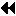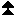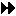Profiler implementation

## Profiler implementationUp: Examples Next: MPI library implementation Previous: Examples

Suppose that the profiler wishes to accumulate the total amount of data sent by the MPI_SEND function, along with the total elapsed time spent in the function. This could trivially be achieved thus

```static int totalBytes;
static double totalTime;

int MPI_SEND(void * buffer, const int count, MPI_Datatype datatype,
int dest, int tag, MPI_comm comm)
{
double tstart = MPI_Wtime();       /* Pass on all the arguments */
int extent;
int result    = PMPI_Send(buffer,count,datatype,dest,tag,comm);

MPI_Type_size(datatype, &extent);  /* Compute size */
totalBytes += count*extent;

totalTime  += MPI_Wtime() - tstart;         /* and time          */

return result;
}
```Up: Examples Next: MPI library implementation Previous: Examples